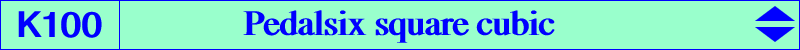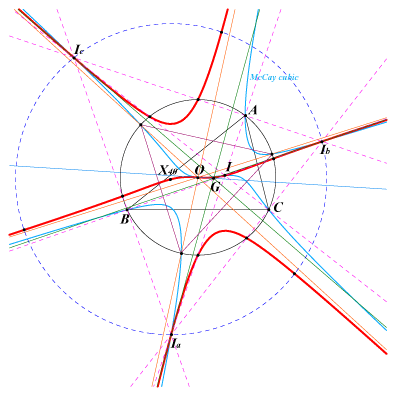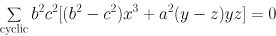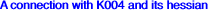X(1), X(3), X(40), X(1670), X(1671) excenters and their reflections in X(3) 6 "second" intersections of the bisectors and the circumcircle called vertices of the two circumperp triangles in TCCT. These points lie on the perpendicular bisectors of ABC. points at infinity of the McCay cubic 7 seven fixed points of a mapping mentioned at K1065Denote by PaPbPc the pedal triangle of point P and by T1, T2, T3, T4, T5, T6 the areas of triangles PBPa, PPaC, PCPb, PPbA, PAPc, PPcB respectively. The locus of point P such that T1^2+T3^2+T5^2 = T2^2+T4^2+T6^2 is K100, a K60++ i.e. a central stelloid with radial center O called pedalsix square cubic. It has three real asymptotes parallel to those of the McCay cubic i.e. perpendicular at O to the sidelines of the Morley triangle. The tangents at the in/excenters concur at X(2). The inflexional tangent at O is the Euler line. K100 is the Musselman (first) cubic K026 for the excentral triangle IaIbIc. K077, K078, K100, K258 are members of a same pencil of cubics generated by the McCay cubic K003 and the decomposed cubic which is the union of the Stammler hyperbola and the line at infinity. See Table 63. See the very similar cubic K1271 and the related septic Q082. K100 is the Laplacian of the quintic Q068 i.e. the locus of points whose polar conic in the quintic is a rectangular hyperbola. The Hessian of K100 is the central focal cubic K1294.The Darboux cubic K004 and its hessian H004 share the same orthic line namely the Euler line. Thus, for any point P on the Euler line, the polar conics of P with respect to K004 and H004 are both rectangular hyperbolas intersecting at Q1, Q2, Q3, Q4. The polar conic of P in K004 passes through X(1670), X(1671), X(3413), X(3414) and its center lies on the line X(3), X(76), X(98), X(99), etc. It is therefore homothetic to the Kiepert hyperbola. It belongs to the pencil generated by the Wallace (diagonal) hyperbola and the union of the line at infinity and the Brocard axis. The polar conic of P in H004 passes through the infinite points of the circum-conic with perspector X(11176) and its center lies on the line passing through X(3) and X(9150). It belongs to a pencil containing the diagonal rectangular hyperbola passing through X(798), X(2234), the in/excenters of ABC and the decomposed conic which is the union of the line at infinity with the line through X(3), X(5106), X(12525). When P traverses the Euler line, the locus of these four points Q1, Q2, Q3, Q4 is the union of the line at infinity and K100. Special cases : • with P = X(20), the hyperbolas are diagonal conics intersecting at the in/excenters. • with P = O, both hyperbolas split into the line at infinity and a line passing through O as seen above. *** See Q002, Q083 for analogous properties when K004 is replaced with K003, K006 respectively.